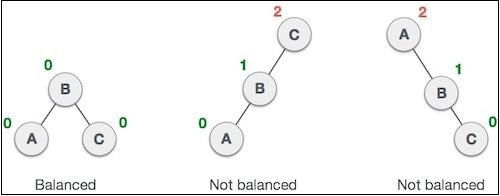# Calculating the balance factor in a Javascript AVL Tree

JavascriptWeb DevelopmentFront End Technology

AVL tree checks the height of the left and the right sub-trees and assures that the difference is not more than 1. This difference is called the Balance Factor.

For example, in the following trees, the first tree is balanced and the next two trees are not balanced −In the second tree, the left subtree of C has height 2 and the right subtree has height 0, so the difference is 2. In the third tree, the right subtree of A has height 2 and the left is missing, so it is 0, and the difference is 2 again. AVL tree permits difference (balance factor) to be only 1.

BalanceFactor = height(left-sutree) − height(right-sutree)

If the difference in the height of left and right sub-trees is more than 1, the tree is balanced using some rotation techniques.

Let us define this method and initialize the class as well −

## Example

class AVLTree {
constructor() {
// Initialize a root element to null.
this.root = null;
}
getBalanceFactor(root) {
return this.getHeight(root.left) - this.getHeight(root.right);
}
getHeight(root) {
let height = 0;
if (root === null) {
height = -1;
} else {
height = Math.max(this.getHeight(root.left), this.getHeight(root.right)) + 1;
}
return height;
}
}
AVLTree.prototype.Node = class {
constructor(data, left = null, right = null) {
this.data = data;
this.left = left;
this.right = right;
}
};